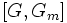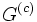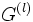# Nilpotent implies solvable

DIRECT: The fact or result stated in this article has a trivial/direct/straightforward proof provided we use the correct definitions of the terms involved
View other results with direct proofs
VIEW FACTS USING THIS: directly | directly or indirectly, upto two steps | directly or indirectly, upto three steps|
This article gives the statement and possibly, proof, of an implication relation between two group properties. That is, it states that every group satisfying the first group property must also satisfy the second group property
View all group property implications | View all group property non-implications
|

## Statement

### Verbal statement

Any nilpotent group is a solvable group. Further, the solvable length is bounded from above by the nilpotence class.

### Property-theoretic statement

The group property of being a nilpotent group is stronger than, or implies, the group property of beign a solvable group.

## Definitions used

### Nilpotent group

Further information: Nilpotent group

A group is said to be a nilpotent group if its lower central series terminates in finitely many steps at the trivial group. The lower central series is here defined as follows:$G_1 = G, G_i = [G,G_{i-1}]$

here$[H,K]$ is the subgroup generated by all commutators between elements of$H$ and elements of$K$.

Note: This is the definition of nilpotent group that is convenient for proving this implication. The other definition, in terms of upper central series, is not convenient for our purpose.

### Solvable group

Further information: Solvable group

A group is said to be a solvable group if its derived series terminate in finitely many steps at the trivial group. The derived series is defined as:$G^{(1)} = [G,G], [itex]G^{(n)} = [G^{(n-1)},G^{(n-1)}$

Note: This is the most convenient definition here.

## Related facts

### Stronger facts

The solvable length of a nilpotent group is not just bounded by the nilpotence class; it is also bounded by the logarithm of the nilpotence class:

## Proof

### Proof using lower central series and derived series

Given: A nilpotent group$G$ with nilpotence class$c$.

To prove:$G$ is solvable with solvable length at most$c$.

Proof: The crucial fact used in the proof is the following lemma: For any group, the$i^{th}$ member of the derived series is contained in the$(i+1)^{th}$ member of the lower central series. We prove this lemma inductively.

Induction base case$i=0$:$G^{(0)} = G = G_1$. Thus,$G^{(0)} \le G_1$.

Induction step: Suppose$G^{(m)} \le G_{m+1}$. Then we have:$G_{m+2} = [G,G_{m+1}]; G^{(m+1)} = [G^{(m)},G^{(m)}]$

Now,$G^{(m)} \le G$ and$G^{(m)} \le G_{m+1}$ (using the induction assumption). Thus, every commutator between$G^{(m)}$ and$G^{(m)}$ is also a commutator between$G$ and$G_{m+1}$. Thus, we have a generating set for$[G^{(m)},G^{(m)}]$ which is a subset of a generating set for$[G,G_m]$.

From this, it follows that$[G^{(m)},G^{(m)}]$ is a subgroup of$[G,G_{m+1}]$. Thus:$G^{(m+1)} \le G_{m+2}$.

This completes the induction.$G^{(c)} \le G_{c+1}$

Since$G$ has class$c$,$G_{c+1}$ is trivial, and hence$G^{(c)}$ is trivial. This means that$G$ is solvable. Further, the smallest$l$ such that$G^{(l)}$ is trivial is at most$c$. So the solvable length is at most equal to the nilpotence class.